# Lesson#19: How to convert digit into word in excel

In this lesson we will learn a method to convert digit into word. I will use functions like CONCATENATE, VLOOKUP, SUM, IF, MOD, which were discussed in my previous posts.

1st Step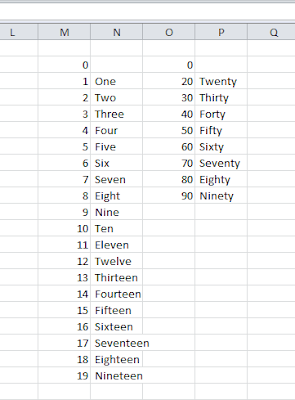I have made a list of relevant word phrase from 0 to 19 and 20, 30, 40, 50, 60, 70, 80, 90. I have intentionally kept the zero blank because we don’t spell 0 as “Zero” when converting into word, it is kept silent.
2nd Step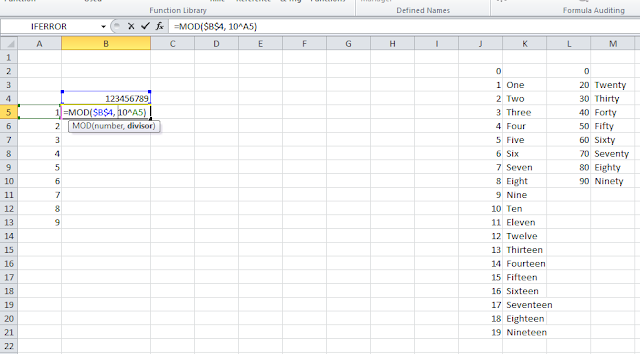For example I have taken the number 123456789 and I am making a chart. In first column I have put 1 to 9. In second column I have added the formula at cell B5 =MOD(\$B\$4, 10^A5) beside 1 which will show the remainder after diving the number by 10^1.

3rd Step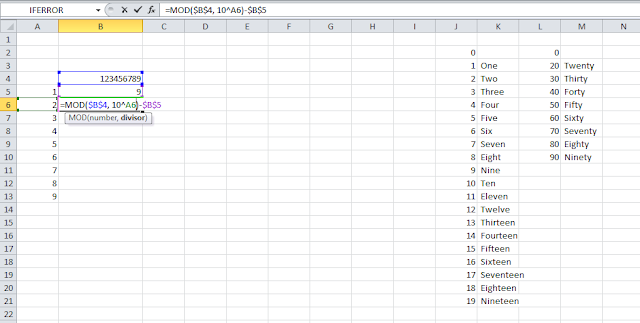At next cell B6 =MOD(\$B\$4, 10^A6)-\$B\$5 beside 2 which will show the result of subtraction between remainder after diving the number by 10^2 and B5.

4th Step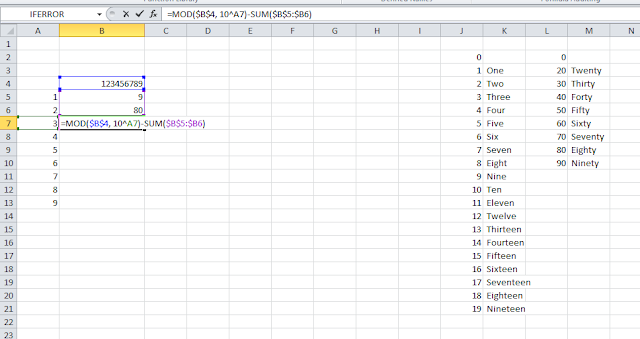At next cell B7 =MOD(\$B\$4, 10^A7)-SUM(\$B\$5:\$B6) beside 3 which will show the result of subtraction between remainder after diving the number by 10^3 and sum of previous cell to B5.

5th Step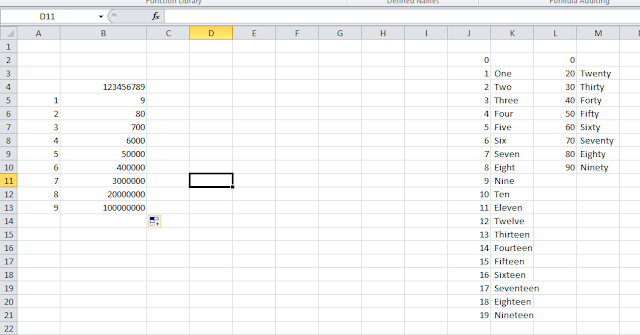I have dragged the formula up to 9th cell.

6th Step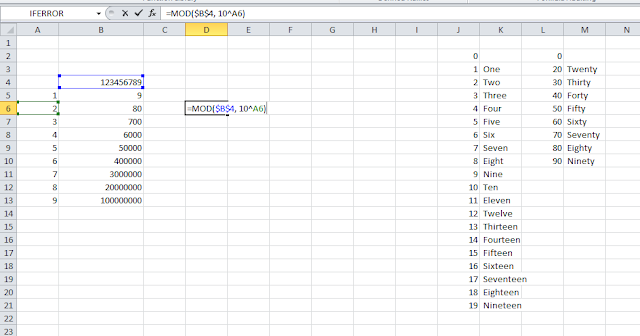At the cell D6 I am putting the formula =MOD(\$B\$4, 10^A6) or =MOD(\$B\$4, 100)which will show the remainder after dividing the number by 10^2 or 100.

7th Step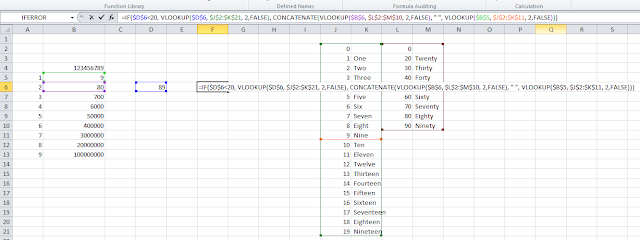Now I will input the logic when the D6 is less than 20 it will lookup the phrase for 0 to 19 and when it will be equal to 20 or larger then it will combine the lookup value for B6 and B5. This is the formula =IF(\$D\$6<20, VLOOKUP(\$D\$6, \$J\$2:\$K\$21, 2,FALSE), CONCATENATE(VLOOKUP(\$B\$6, \$L\$2:\$M\$10, 2,FALSE), ” “, VLOOKUP(\$B\$5, \$J\$2:\$K\$11, 2,FALSE)))

8th Step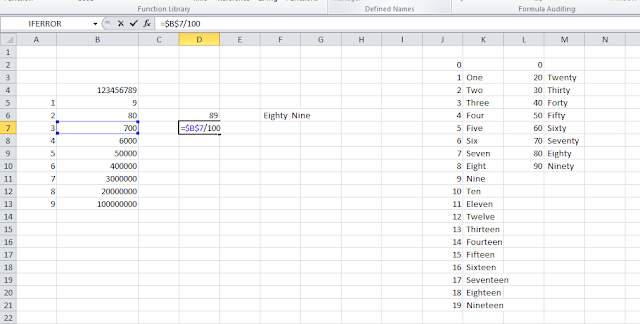Next step will be for Hundred. At D7 I will put the value of =\$B\$7/100.

9th Step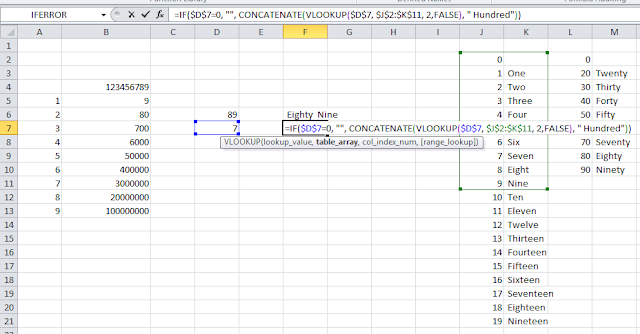For spelling it I will put the formula =IF(\$D\$7=0, “”, CONCATENATE(VLOOKUP(\$D\$7, \$J\$2:\$K\$11, 2,FALSE), ” Hundred”))

10th Step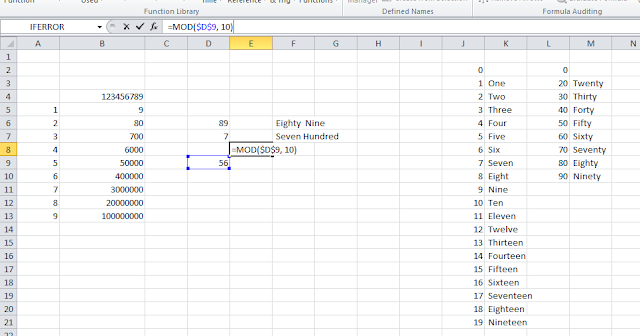For spelling the Thousand portion I have to extract the two digit number by using =(MOD(\$B\$4, 100000)-SUM(\$B\$5:\$B\$7))/1000. At E8  I have put the formula =MOD(\$D\$9, 10)

11th Step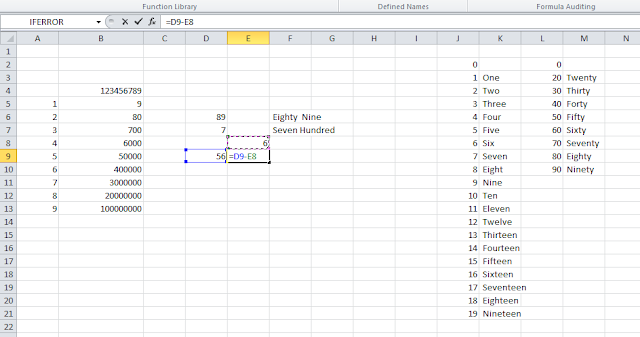At E9 I have put the formula =D9-E8.

12th Step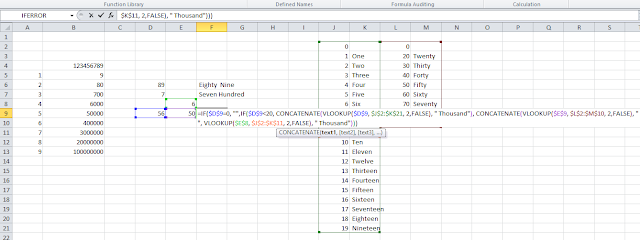Now the logic is if the value of D9 is less than 20 then it will lookup the phrase for 0 to 19 chart and add “Thousand” as suffix, else it will concatenate the phrase for E9 and E8 then will add “Thousand” as suffix. The formula is =IF(\$D\$9=0, “”,IF(\$D\$9<20, CONCATENATE(VLOOKUP(\$D\$9, \$J\$2:\$K\$21, 2,FALSE), ” Thousand”), CONCATENATE(VLOOKUP(\$E\$9, \$L\$2:\$M\$10, 2,FALSE), ” “, VLOOKUP(\$E\$8, \$J\$2:\$K\$11, 2,FALSE), ” Thousand”))). When D9 will be zero then it will be silent.

13th Step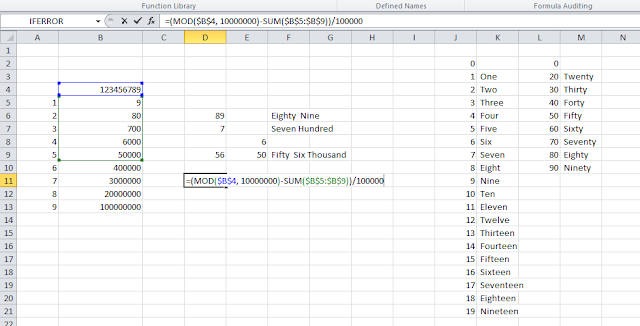Like the previous steps for spelling Thousand I will put formula for Lakhs. For spelling the Lakh portion I have to extract the two digit number by using =(MOD(\$B\$4, 10000000)-SUM(\$B\$5:\$B\$9))/100000.

14th Step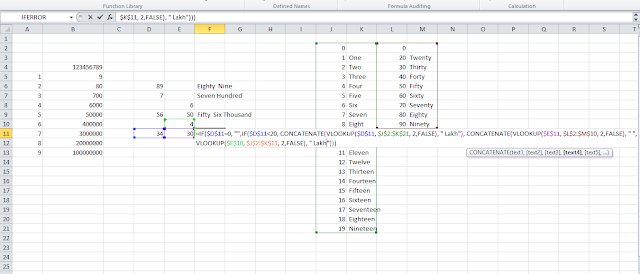Now the logic is if the value of D11 is less than 20 then it will lookup the phrase for 0 to 19 chart and add “Lakh” as suffix, else it will concatenate the phrase for E11 and E10 then will add “Lakh” as suffix. The formula is =IF(\$D\$11=0, “”,IF(\$D\$11<20, CONCATENATE(VLOOKUP(\$D\$11, \$J\$2:\$K\$21, 2,FALSE), ” Lakh”), CONCATENATE(VLOOKUP(\$E\$11, \$L\$2:\$M\$10, 2,FALSE), ” “, VLOOKUP(\$E\$10, \$J\$2:\$K\$11, 2,FALSE), ” Lakh”))). When D11 will be zero then it will be silent.

15th Step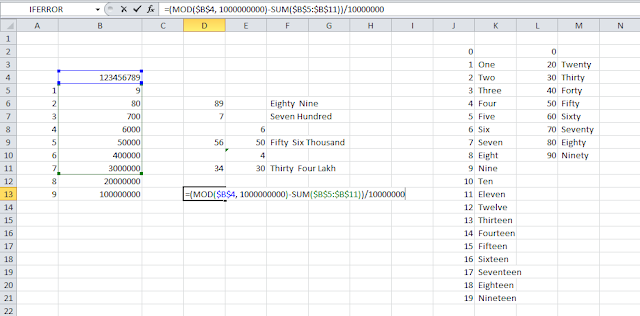Like the previous steps for spelling Lakh I will put formula for Crore. For spelling the Crore portion I have to extract the two digit number by using =(MOD(\$B\$4, 1000000000)-SUM(\$B\$5:\$B\$11))/10000000.
16th Step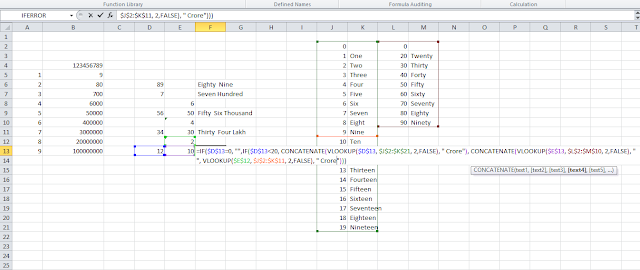Now the logic is if the value of D13 is less than 20 then it will lookup the phrase for 0 to 19 chart and add “Crore” as suffix, else it will concatenate the phrase for E13 and E12 then will add “Crore” as suffix. The formula is =IF(\$D\$13=0, “”,IF(\$D\$13<20, CONCATENATE(VLOOKUP(\$D\$13, \$J\$2:\$K\$21, 2,FALSE), ” Crore”), CONCATENATE(VLOOKUP(\$E\$13, \$L\$2:\$M\$10, 2,FALSE), ” “, VLOOKUP(\$E\$12, \$J\$2:\$K\$11, 2,FALSE), ” Crore”))). When D13 will be zero then it will be silent.
17th Step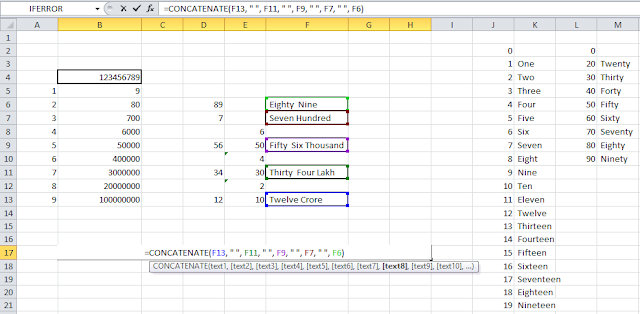For spelling it completely I will use CONCETANATE function like I have serehown in picture. The formula will be =CONCATENATE(F13, ” “, F11, ” “, F9, ” “, F7, ” “, F6).
Now the result is here in this picture.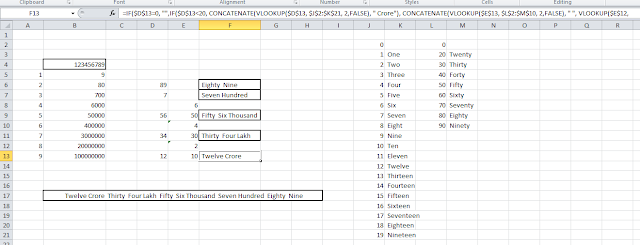Here I have given download link of the file for my readers where another file is available which converts currency to word.1.express vpn keys 2018 says: